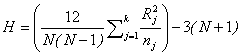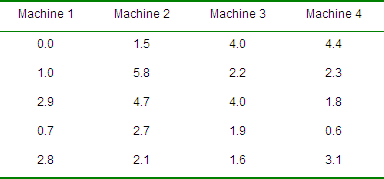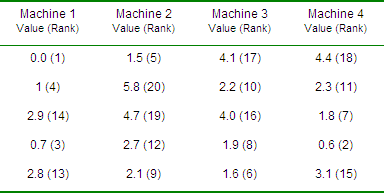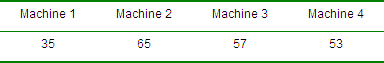# The Kruskal-Wallis (KW) Test

The Kruskal-Wallis (KW) test is a non-parametric test used to compare three or more independent groups of data. It is used to test the null hypothesis that all populations have identical distribution functions against the alternative hypothesis that at least two of the samples differ. Specifically, InfinityQS SPC uses KW to test for differences in the variability among multiple groups of data. That is, the KW test is only performed on data contained in the charts that track variability (moving-range, range and sigma charts).

Unlike the parametric independent group ANOVA (one way ANOVA), this non-parametric test makes no assumptions about the distribution of the data. This, like many non-parametric tests, uses the ranks of the data rather than their raw values to calculate the statistic. Since this test does not make a distributional assumption, it is not as powerful as the ANOVA.

The test statistic for the Kruskal-Wallis test is H. This value is compared to a table of critical values (X2 table). If H exceeds the critical value for H at some significance level (InfinityQS uses 0.01) it means that there is evidence to believe that at least one data group's level of variability is significantly different than the others.Where:

k = number of independent groups
N = total number of values across all groups
n = total number of values within the specific group
j = group number
R = sum of the ranks from the jth group

### KW Calculation Example

Let's say that we have a dataset with the following range values:Next, we rank each value from 1 to 20:Summing the ranked values:Substituting into the formula:Looking up 2.76 on the Chi-Square table d.f. = k-1 = 4-1 = 3

In this case, the p value is > 0.01, therefore, InfinityQS will display an (Accept). Meaning, one cannot detect a difference between the machine's variability. InfinityQS will display the actual p value.

Need help?
​For further information contact GetInTouch@infinityqs.com
Toll Free: 1.800.772.7978​

### Take the first step from quality to excellence

Take the Next Steps